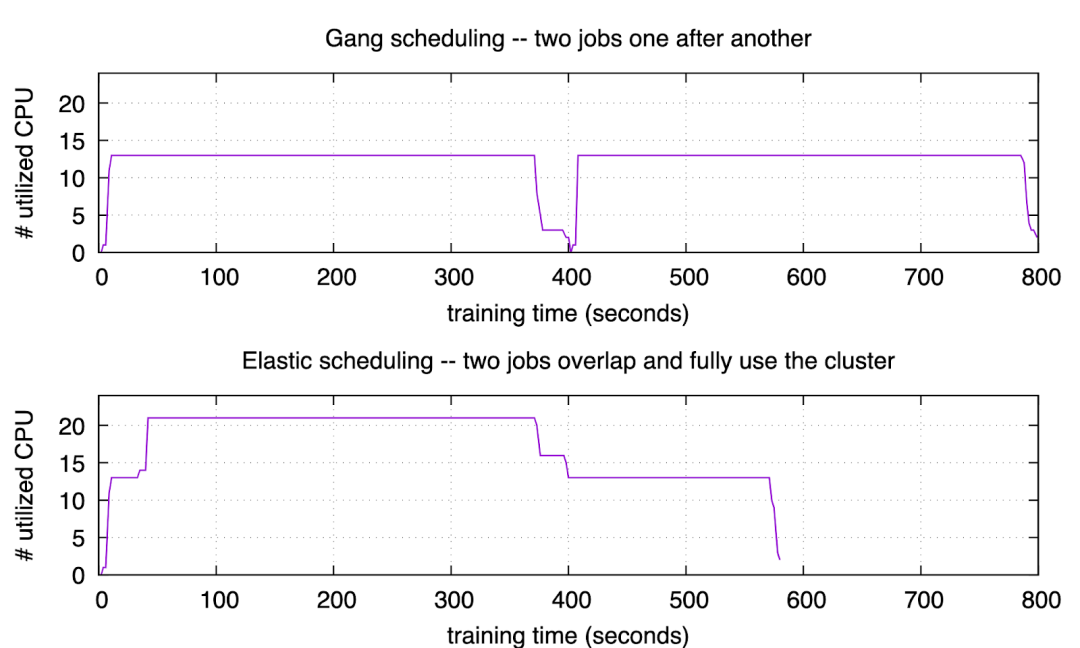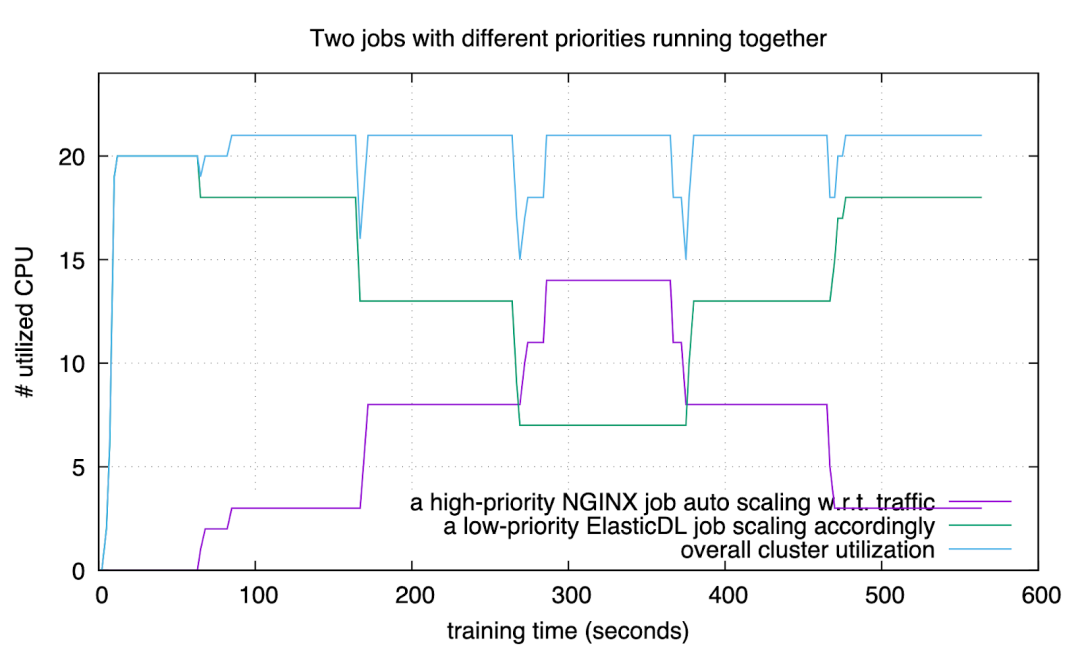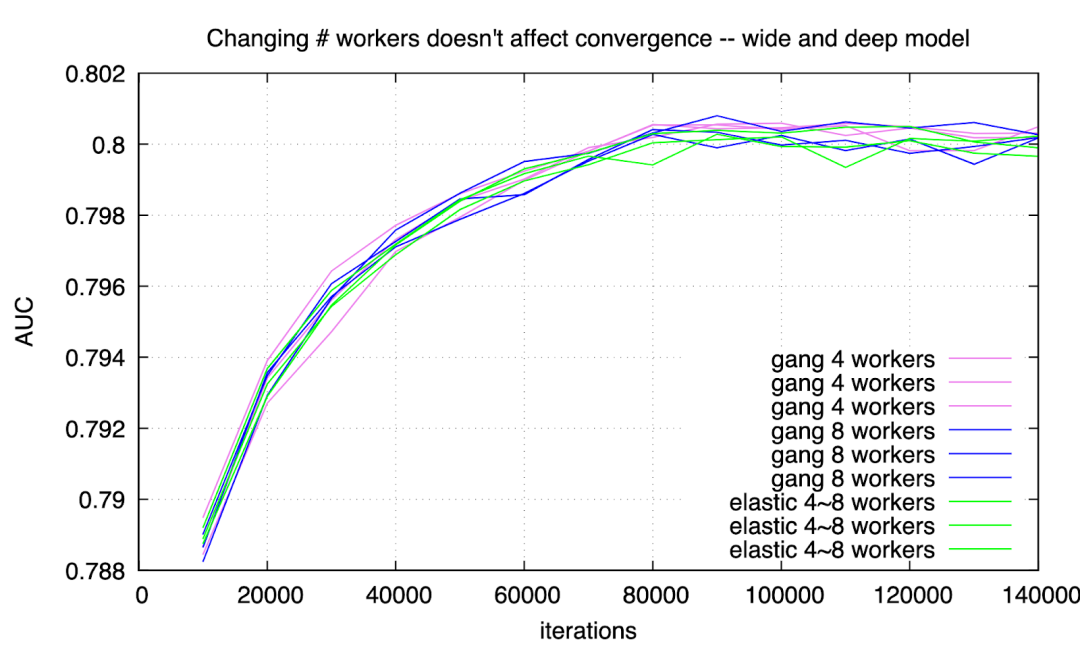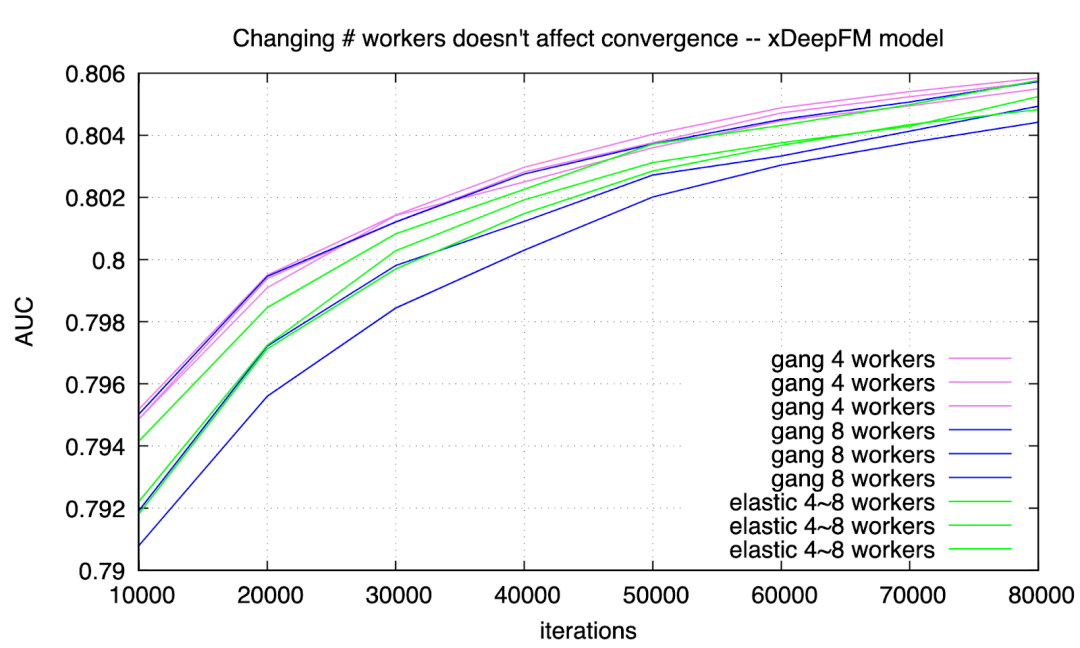# 支付宝如何优化深度学习集群，解决两大痛点？

2020/07/13 16:48

ElasticDL 是一个基于 TensorFlow 2.x 和 Kubernetes 的开源的分布式深度学习编程框架。2019 年秋天的 Google Developer Day 活动中来自蚂蚁金服的 ElasticDL 团队展示了 ElasticDL 的第一个开源版本。本文更新这大半年来 ElasticDL 项目的进展，尤其是性能优化和业务落地。

• ElasticDL
https://github.com/sql-machine-learning/elasticdl

ElasticDL 的首要设计意图是简化分布式编程。它允许用户只提供用 TensorFlow 2.0 API 描述的模型，而不需要用户写分布式训练过程代码。用户的模型定义只要能在本地调通，即可在分布式环境下用大规模数据训练模型，从而提升研发效率。

• TensorFlow Estimator 作为构建在 TensorFlow 之上的一层 API，允许用户只需定义模 型，而训练过程封装在一个函数调用里。利用 Kubeflow 提供的 TF operator，我们可以将该训练过程以分布式作业的方式启动在Kubernetes 上。这个方案的局限是：它仅支持 TensorFlow 的 graph mode，不支持 eager execution；而 eager execution 可以大幅简化调试，尤其方便跟踪网络各层输出。
• Keras API 支持 TensorFlow 2.x 和 eager execution。目前 TensorFlow 2.x Keras API 还暂不支持 ParameterServer 分布式策略，对 AllReduce 分布式策略提供了实验性的支持。
• Horovod 对用户代码有侵入性，用户除了必须熟悉 TensorFlow API 之外，还需学习 Horovod API。

Estimator TensorFlow Estimator API Kubeflow TF-operator
Keras TensorFlow Keras API Kubeflow TF-operator
Horovod Horovod with TensorFlow Kubeflow MPI-operator
ElasticDL TensorFlow Keras API ElasticDL master process per job

def forward():    inputs = tf.keras.Input(shape=(28, 28), name="image")    x = tf.keras.layers.Reshape((28, 28, 1))(inputs)    x = tf.keras.layers.Conv2D(32, kernel_size=(3, 3), activation="relu")(x)    x = tf.keras.layers.Conv2D(64, kernel_size=(3, 3), activation="relu")(x)    x = tf.keras.layers.BatchNormalization()(x)    x = tf.keras.layers.MaxPooling2D(pool_size=(2, 2))(x)    x = tf.keras.layers.Dropout(0.25)(x)    x = tf.keras.layers.Flatten()(x)    outputs = tf.keras.layers.Dense(10)(x)    return tf.keras.Model(inputs=inputs, outputs=outputs, name="mnist_model")

def loss(labels, predictions):    labels = tf.reshape(labels, [-1])    return tf.reduce_mean(        input_tensor=tf.nn.sparse_softmax_cross_entropy_with_logits(            logits=predictions, labels=labels        )    )def optimizer(lr=0.1):    return tf.optimizers.SGD(lr)def feed(dataset, mode, _):    def _parse_data(record):        if mode == Mode.PREDICTION:            feature_description = {                "image": tf.io.FixedLenFeature([28, 28], tf.float32)            }        else:            feature_description = {                "image": tf.io.FixedLenFeature([28, 28], tf.float32),                "label": tf.io.FixedLenFeature(, tf.int64),            }        r = tf.io.parse_single_example(record, feature_description)        features = {            "image": tf.math.divide(tf.cast(r["image"], tf.float32), 255.0)        }        if mode == Mode.PREDICTION:            return features        else:            return features, tf.cast(r["label"], tf.int32)    dataset = dataset.map(_parse_data)    if mode == Mode.TRAINING:        dataset = dataset.shuffle(buffer_size=1024)    return dataset

ElasticDL 的弹性训练过程

Master 负责动态数据划分

ElasticDL 的动态数据划分是基于索引的。ElasticDL 要求训练数据是一个或者多个 RecordIO 格式的文件，或者是 MaxCompute 数据库系统中的表 (table)。这两种数据源都允许 master 进程在开始训练之前，在基本存储单元 (block) 间快速跳跃着扫描数据，把数据分成小段，称之为任务 (task)。每个 task 包括的内容如下：
1. 文件名或者表名，
2. 第一条记录相对于文件（或者表）开始处的偏移 (offset)，
• RecordIO
https://github.com/wangkuiyi/recordio
• MaxCompute
https://www.alibabacloud.com/zh/product/maxcompute

Worker 调用 TensorFlow Eager Execution

1. 读取一个 mini-batch 的训练数据。
2. 用本地模型 (local model) 作为参数调用用户定义的 forward 函数以计算 cost。如果 模型很大，则部分参数可能来自于 parameter server。
3. 给定 cost，worker 利用 TensorFlow eager execution 的 GradientTape 机制，进行 backward 计算，得到梯度 (gradient)。
4. 如果是 synchronous SGD，此时 worker 调用 AllReduce 实现 FTlib 来同步 gradients 并且更新模型。如果是 asynchronous SGD，worker 不定时的向 parameter server 上传 gradients，也不定时地从 parameter server 获取全局模型参数。

• 在 parameter server 上惰性初始化 (lazy initialize) embedding vectors —— 在使用到 vector 的时候才初始化。
• 把一个 embedding table 拆分到多个 parameter server 进程里以均衡存储与通信负载。
• worker 从 PS 请求 embedding vectors 时，先滤除重复的 embedding ID，只取回不同 ID 的 vectors，从而减少通信量。
• worker 向 PS 发送梯度时，先把相同 ID 的梯度进行合并（调用 TensorFlow 的 embedding vector combination 函数），从而减少通信量。

ElasticDL 实现的弹性调度和刚性调度 ( Gang Scheduling ) 是对应的。刚性调度的简洁不求甚解的描述是：一个作业里的 n 个进程，运行时如果有一个进程挂了（比如被更高优先级的作业抢占了资源），则整个作业挂掉。等资源足够再启动所有的 n 个进程了， 则可以重启（或者从最近的 checkpoint 恢复）。

• Gang Scheduling
https://en.wikipedia.org/wiki/Gang_schedulingFigure 1: overlap jobs

• 用户等待作业启动时间几乎是 0。这对于 AI 工作很重要，因为用户最关注的是第一个迭代尽快开始—— 如果第一个迭代失败了，很可能是用户程序的 bug。另外，深度学习模型往往需要手动调优，学习率、optimizer、activation 等配置如果不合理，往往在前几个迭代就能发现；因此第一个迭代能立刻开始，对模型调优的工作效率提高有很大帮助。
• 集群利用率高。第二个实验 (elastic scheduling) 执行期间，有一段时间集群利用率是 100%；其他时间也不低于第一个实验 (gang scheduling)。
• 作业完成更快。第二个试验里，两个作业用了约 580 秒；第一个实验里需要约 795 秒。Figure 2: auto reactFigure 3: wide-n-deep training convergesFigure 4: xdeepfm training converges

ElasticDL 的设计和实现依托了 TensorFlow 2.x 提供的高效率的模型描述 API。也依赖了 TensorFlow eager execution 提供的 GradientTape 机制 —— 使得 ElasticDL 可以在不改变 TensorFlow runtime 的情况下，结合 Kubernetes 实现彻底的弹性调度（进程数可增也可减），从而实现了减少作业启动 的等待时间，提升集群利用率，和提升研发效率的效果。

END -0
0 收藏

### 作者的其它热门文章0 评论
0 收藏
0